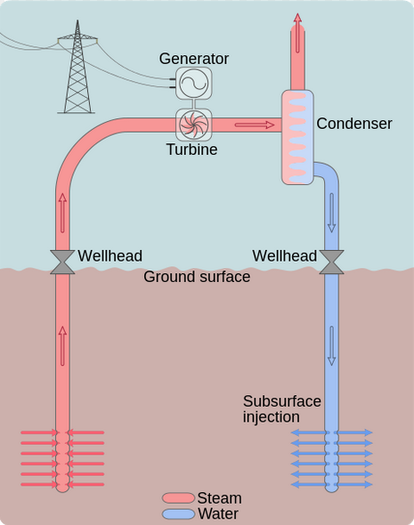# MAximum Heat Engine Efficiency

The change in entropy for a constant-temperature process can be calculated from the heat transferred (Q) and the temperature at which the transfer occurs (T) as:

(1)Notice that we definitely need to use the absolute temperature scale when working with the change in equation, or else we might find ourselves attempting to divide by zero! With regard to the Second Law of Thermodynamics, we know that for any real process the totalmust always be greater than zero.

### Everyday Example: Geothermal Heat Engine

Let’s imagine molten rock from the Earth’s mantle pushes partway through the Earth’s , and keeps a region of at a constant temperature of 300 °C . If the rock was not too deep, we could install pipes in the rock, and then boil water by running it through the pipes. We would basically have a giant pressure cooker! Rather than cook food, we could release the pressurized steam to push on a piston or spin a turbine.  After releasing the pressure and getting some work out, we would be left with lower pressure steam. We could condense the steam back to water by running it through pipes exposed to the 20 °C air above ground. Thermal energywould transfer from the steam to the air as exhaust heat, the steam would condense into liquid water, and we could start again.The dry steam cycle from “Geothermal power plants” by Energy Education, University of Calgary

Machines like the one described that convert thermal energy into mechanical energy are called a heat engines.  Your car is powered by an internal combustion heat engine.  Let’s see how and the Second Law of Thermodynamics determine the efficiency of our geothermal heat engine.

First we calculate the entropy change when 1000 J of thermal energy transfers out of the rock to the water to run the engine, remembering to convert the 300 °C rock temperature to by adding 273 K:If our engine is real, then so are its processes,  which means that running the engine must increase the total of the universe, according to the Second Law of Thermodynamics. We need to find out how much thermal energy must be transferred from the low pressure steam into the air at 20 °C (293 K) in order for the entropy of the air to increase by at least as much as the rock entropy decreased (1.75 J/K). We can find this by rearranging the change in entropy equation and inserting a positive entropy change that is equal in size to the the negative change experienced by the hot rock:Transferring the 513 J of thermal energy into the air leaves only 487 J of the original 1000 J input energy available for doing . Therefore the maximum possible efficiency of our engine is limited, no matter how well designed, even if all mechanical inefficiencies like could some how be eliminated. The maximum theoretical efficiency is:Multiplying by 100 would give us the efficiency as a percentage: 49 %. This is a maximum possible efficiency. Any engine we actually built would be less efficient.

The First Law of Thermodynamics told us that you cannot build an engine that is more than 100 % efficient because energy cannot be created. Even worse, the Second Law tells us that even if we managed to eliminate all mechanical inefficiencies, such as , we still can’t get up to 100 % because all engines must exhaust some energy in order to increase entropy overall. The theoretical maximum efficiency, which is always less than 100 %, is known as the (ec) and depends only on the high and low operating temperatures (TH and TL), as we saw in the previous example.  The formula for the Carnot Efficiency (ec) effectively recreates all of the work we did in the previous example:

(2)The theoretical engine which could actually produce that theoretically maximum efficiency is known as the Carnot Engine. The operating principles of the Carnot Engine are well known, having been developed by Nicolas Léonard Sadi Carnot in 1824, but the engine cannot be realistically designed or built.

### Reinforcement Exercise: Carnot Efficiency

First check that the Carnot Efficiency equation above gives the correct maximum efficiency for our example geothermal heat engine, then do the problem below.

You will find that this engine is more efficient just because the hot  operating temperature higher, even though it still works in the same way and nothing else has changed. The efficiency increased because the input energy started out more concentrated and less dispersed (indicated by higher temperature), so less of that energy had to become dispersed into the environment in order to ensure that entropy increased by a sufficient amount to satisfy the Second Law of Thermodynamics. For this reason, thermal energy that starts out more concentrated (at high temperature) is said to be  higher quality energy.

### Reinforcement Exercise: